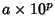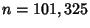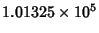## Scientific Notation

Scientific notation is the expression of a numberin the form, whereis the Floor of the base-10 Logarithm of(the order of magnitude''), andis a Real Number satisfying. For example, in scientific notation, the numberhas order of magnitudesowould be written. The special case of 0 does not have a unique representation in scientific notation, i.e.,.